Main Content

# pdeplot

Plot solution or mesh for 2-D problem

## Syntax

``pdeplot(model,'XYData',results.NodalSolution)``
``pdeplot(model,'XYData',results.Temperature,'ColorMap','hot')``
``pdeplot(model,'XYData',results.VonMisesStress,'Deformation',results.Displacement)``
``pdeplot(model,'XYData',results.ModeShapes.ux)``
``pdeplot(model,'XYData',results.ElectricPotential)``
``pdeplot(model)``
``pdeplot(mesh)``
``pdeplot(nodes,elements)``
``pdeplot(p,e,t)``
``pdeplot(___,Name,Value)``
``h = pdeplot(___)``

## Description

example

````pdeplot(model,'XYData',results.NodalSolution)` plots the solution of a `model` at nodal locations as a colored surface plot using the default `'jet'` colormap.```

example

````pdeplot(model,'XYData',results.Temperature,'ColorMap','hot')` plots the temperature at nodal locations for a 2-D thermal analysis model. This syntax creates a colored surface plot using the `'hot'` colormap.```

example

````pdeplot(model,'XYData',results.VonMisesStress,'Deformation',results.Displacement)` plots the von Mises stress and shows the deformed shape for a 2-D structural analysis model.```

example

````pdeplot(model,'XYData',results.ModeShapes.ux)` plots the `x`-component of the modal displacement for a 2-D structural modal analysis model.```

example

````pdeplot(model,'XYData',results.ElectricPotential)` plots the electric potential at nodal locations for a 2-D electrostatic analysis model.```

example

````pdeplot(model)` plots the mesh specified in `model`.```

example

````pdeplot(mesh)` plots the mesh defined as a `Mesh` property of a 2-D `model` object of type `PDEModel`.```

example

````pdeplot(nodes,elements)` plots the mesh defined by its `nodes` and `elements`.```

example

````pdeplot(p,e,t)` plots the mesh described by `p`,`e`, and `t`.```

example

````pdeplot(___,Name,Value)` plots the mesh, the data at the nodal locations, or both the mesh and the data, depending on the `Name,Value` pair arguments. Use any arguments from the previous syntaxes.Specify at least one of the `FlowData` (vector field plot), `XYData` (colored surface plot), or `ZData` (3-D height plot) name-value pairs. Otherwise, `pdeplot` plots the mesh with no data. You can combine any number of plot types.For a thermal model, you can plot temperature or gradient of temperature.For a structural model, you can plot displacement, stress, strain, and von Mises stress. In addition, you can show the deformed shape and specify the scaling factor for the deformation plot.```

example

````h = pdeplot(___)` returns a handle to a plot, using any of the previous syntaxes.```

## Examples

collapse all

Create a PDE model. Include the geometry of the built-in function `lshapeg`. Mesh the geometry and plot it.

```model = createpde; geometryFromEdges(model,@lshapeg); mesh = generateMesh(model); pdeplot(model)```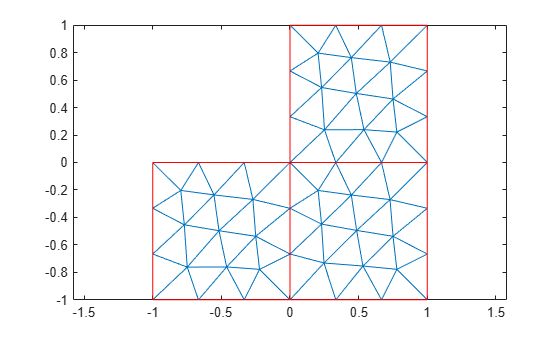Alternatively, you can plot a mesh by using `mesh` as an input argument.

`pdeplot(mesh)`Another approach is to use the nodes and elements of the mesh as input arguments for `pdeplot`.

`pdeplot(mesh.Nodes,mesh.Elements)`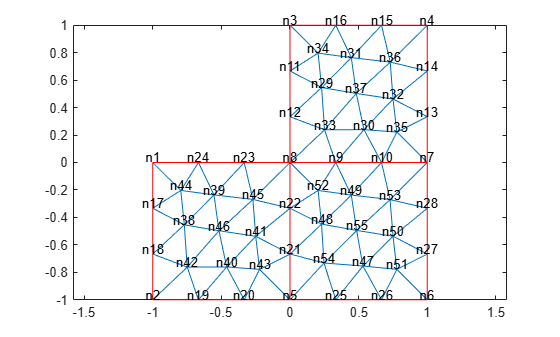Display the node labels. Use `xlim` and `ylim` to zoom in on particular nodes.

```pdeplot(model,'NodeLabels','on') xlim([-0.2,0.2]) ylim([-0.2,0.2])```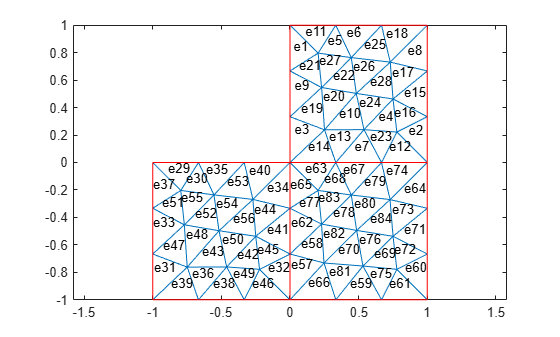Display the element labels.

```pdeplot(model,'ElementLabels','on') xlim([-0.2,0.2]) ylim([-0.2,0.2])```Create colored 2-D and 3-D plots of a solution to a PDE model.

Create a PDE model. Include the geometry of the built-in function `lshapeg`. Mesh the geometry.

```model = createpde; geometryFromEdges(model,@lshapeg); generateMesh(model);```

Set the zero Dirichlet boundary conditions on all edges.

`applyBoundaryCondition(model,'dirichlet','Edge',1:model.Geometry.NumEdges,'u',0);`

Specify the coefficients and solve the PDE.

```specifyCoefficients(model,'m',0, ... 'd',0, ... 'c',1, ... 'a',0, ... 'f',1); results = solvepde(model)```
```results = StationaryResults with properties: NodalSolution: [1177x1 double] XGradients: [1177x1 double] YGradients: [1177x1 double] ZGradients: [] Mesh: [1x1 FEMesh] ```

Access the solution at the nodal locations.

`u = results.NodalSolution;`

Plot the 2-D solution.

`pdeplot(model,'XYData',u)`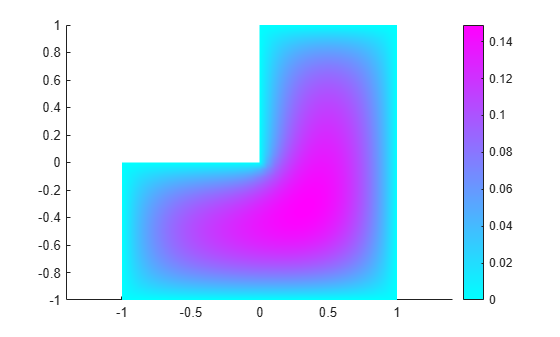Plot the 3-D solution.

`pdeplot(model,'XYData',u,'ZData',u)`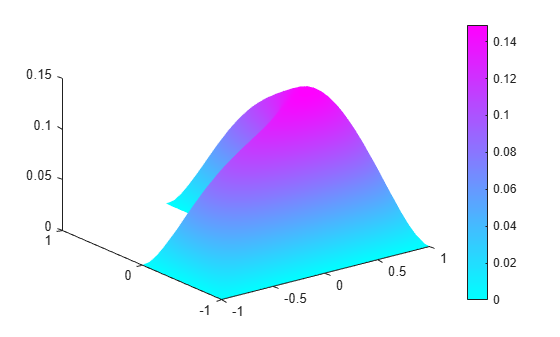Plot the gradient of a PDE solution as a quiver plot.

Create a PDE model. Include the geometry of the built-in function `lshapeg`. Mesh the geometry.

```model = createpde; geometryFromEdges(model,@lshapeg); generateMesh(model);```

Set the zero Dirichlet boundary conditions on all edges.

`applyBoundaryCondition(model,'dirichlet','Edge',1:model.Geometry.NumEdges,'u',0);`

Specify coefficients and solve the PDE.

```specifyCoefficients(model,'m',0, ... 'd',0, ... 'c',1, ... 'a',0, ... 'f',1); results = solvepde(model)```
```results = StationaryResults with properties: NodalSolution: [1177x1 double] XGradients: [1177x1 double] YGradients: [1177x1 double] ZGradients: [] Mesh: [1x1 FEMesh] ```

Access the gradient of the solution at the nodal locations.

```ux = results.XGradients; uy = results.YGradients;```

Plot the gradient as a quiver plot.

`pdeplot(model,'FlowData',[ux,uy])`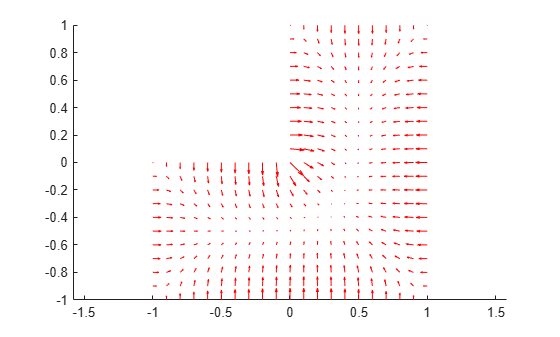Plot the solution of a 2-D PDE in 3-D with the `'jet'` coloring and a mesh, and include a quiver plot. Get handles to the axes objects.

Create a PDE model. Include the geometry of the built-in function `lshapeg`. Mesh the geometry.

```model = createpde; geometryFromEdges(model,@lshapeg); generateMesh(model);```

Set zero Dirichlet boundary conditions on all edges.

`applyBoundaryCondition(model,'dirichlet','Edge',1:model.Geometry.NumEdges,'u',0);`

Specify coefficients and solve the PDE.

```specifyCoefficients(model,'m',0, ... 'd',0, ... 'c',1, ... 'a',0, ... 'f',1); results = solvepde(model)```
```results = StationaryResults with properties: NodalSolution: [1177x1 double] XGradients: [1177x1 double] YGradients: [1177x1 double] ZGradients: [] Mesh: [1x1 FEMesh] ```

Access the solution and its gradient at the nodal locations.

```u = results.NodalSolution; ux = results.XGradients; uy = results.YGradients;```

Plot the solution in 3-D with the `'jet'` coloring and a mesh, and include the gradient as a quiver plot.

```h = pdeplot(model,'XYData',u,'ZData',u, ... 'FaceAlpha',0.5, ... 'FlowData',[ux,uy], ... 'ColorMap','jet', ... 'Mesh','on')```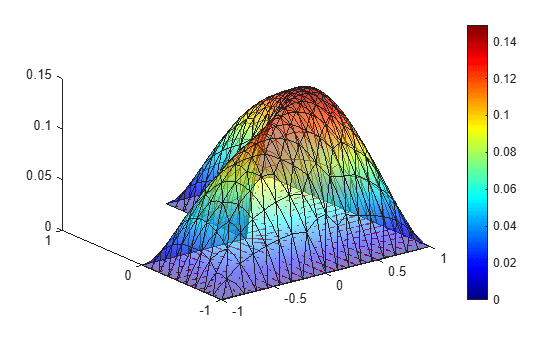```h = 3x1 graphics array: Patch Quiver ColorBar ```

Solve a 2-D transient thermal problem.

Create a transient thermal model for this problem.

`thermalmodel = createpde('thermal','transient');`

Create the geometry and include it in the model.

```SQ1 = [3; 4; 0; 3; 3; 0; 0; 0; 3; 3]; D1 = [2; 4; 0.5; 1.5; 2.5; 1.5; 1.5; 0.5; 1.5; 2.5]; gd = [SQ1 D1]; sf = 'SQ1+D1'; ns = char('SQ1','D1'); ns = ns'; dl = decsg(gd,sf,ns); geometryFromEdges(thermalmodel,dl); pdegplot(thermalmodel,'EdgeLabels','on','FaceLabels','on') xlim([-1.5 4.5]) ylim([-0.5 3.5]) axis equal```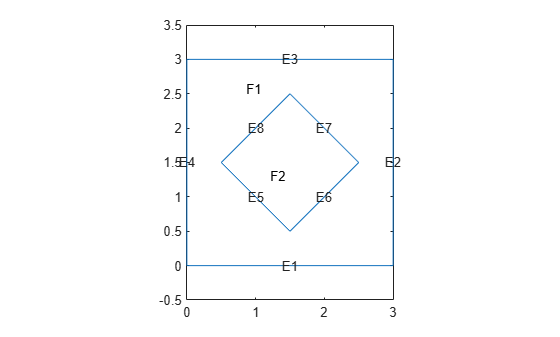For the square region, assign these thermal properties:

• Thermal conductivity is $10\text{\hspace{0.17em}}\mathrm{W}/\left(\mathrm{m}{\cdot }^{\circ }\mathrm{C}\right)$

• Mass density is $2\text{\hspace{0.17em}}\mathrm{kg}/{\mathrm{m}}^{3}$

• Specific heat is $0.1\text{\hspace{0.17em}}\mathrm{J}/\left({\mathrm{kg}\cdot }^{\circ }\mathrm{C}\right)$

```thermalProperties(thermalmodel,'ThermalConductivity',10, ... 'MassDensity',2, ... 'SpecificHeat',0.1, ... 'Face',1);```

For the diamond region, assign these thermal properties:

• Thermal conductivity is $2\text{\hspace{0.17em}}\mathrm{W}/\left(\mathrm{m}{\cdot }^{\circ }\mathrm{C}\right)$

• Mass density is $1\text{\hspace{0.17em}}\mathrm{kg}/{\mathrm{m}}^{3}$

• Specific heat is $0.1\text{\hspace{0.17em}}\mathrm{J}/\left({\mathrm{kg}\cdot }^{\circ }\mathrm{C}\right)$

```thermalProperties(thermalmodel,'ThermalConductivity',2, ... 'MassDensity',1, ... 'SpecificHeat',0.1, ... 'Face',2);```

Assume that the diamond-shaped region is a heat source with a density of $4\text{\hspace{0.17em}}\mathrm{W}/{\mathrm{m}}^{2}$.

`internalHeatSource(thermalmodel,4,'Face',2);`

Apply a constant temperature of $0{\phantom{\rule{0.16666666666666666em}{0ex}}}^{\circ }C$ to the sides of the square plate.

`thermalBC(thermalmodel,'Temperature',0,'Edge',[1 2 7 8]);`

Set the initial temperature to 0 °C.

`thermalIC(thermalmodel,0);`

Generate the mesh.

`generateMesh(thermalmodel);`

The dynamics for this problem are very fast. The temperature reaches a steady state in about 0.1 seconds. To capture the interesting part of the dynamics, set the solution time to `logspace(-2,-1,10)`. This command returns 10 logarithmically spaced solution times between 0.01 and 0.1.

`tlist = logspace(-2,-1,10);`

Solve the equation.

`thermalresults = solve(thermalmodel,tlist)`
```thermalresults = TransientThermalResults with properties: Temperature: [1481x10 double] SolutionTimes: [1x10 double] XGradients: [1481x10 double] YGradients: [1481x10 double] ZGradients: [] Mesh: [1x1 FEMesh] ```

Plot the solution with isothermal lines by using a contour plot.

```T = thermalresults.Temperature; pdeplot(thermalmodel,'XYData',T(:,10),'Contour','on','ColorMap','hot')```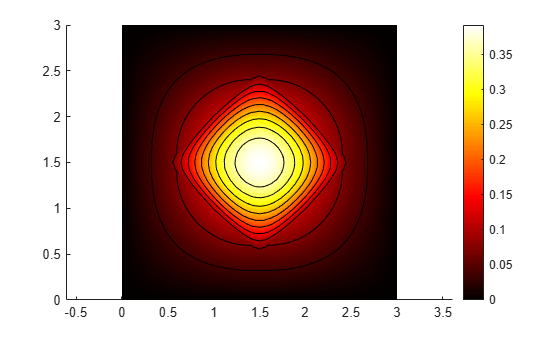Create a structural analysis model for a static plane-strain problem.

`structuralmodel = createpde('structural','static-planestrain');`

Create the geometry and include it in the model. Plot the geometry.

```geometryFromEdges(structuralmodel,@squareg); pdegplot(structuralmodel,'EdgeLabels','on') axis equal```Specify the Young's modulus and Poisson's ratio.

```structuralProperties(structuralmodel,'PoissonsRatio',0.3, ... 'YoungsModulus',210E3);```

Specify the x-component of the enforced displacement for edge 1.

`structuralBC(structuralmodel,'XDisplacement',0.001,'Edge',1);`

Specify that edge 3 is a fixed boundary.

`structuralBC(structuralmodel,'Constraint','fixed','Edge',3);`

Generate a mesh and solve the problem.

```generateMesh(structuralmodel); structuralresults = solve(structuralmodel);```

Plot the deformed shape using the default scale factor. By default, `pdeplot` internally determines the scale factor based on the dimensions of the geometry and the magnitude of deformation.

```pdeplot(structuralmodel,'XYData',structuralresults.VonMisesStress, ... 'Deformation',structuralresults.Displacement, ... 'ColorMap','jet')```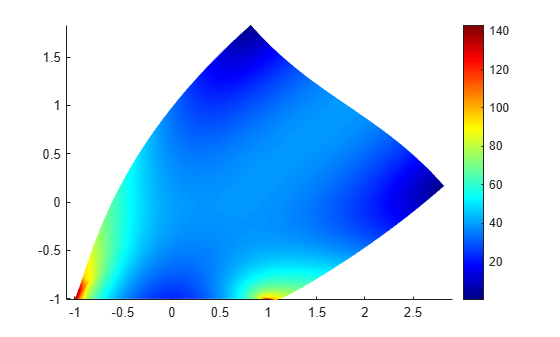Plot the deformed shape with the scale factor 500.

```pdeplot(structuralmodel,'XYData',structuralresults.VonMisesStress, ... 'Deformation',structuralresults.Displacement, ... 'DeformationScaleFactor',500,... 'ColorMap','jet')```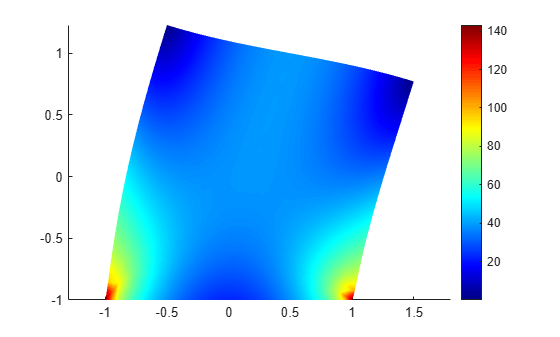Plot the deformed shape without scaling.

```pdeplot(structuralmodel,'XYData',structuralresults.VonMisesStress, ... 'ColorMap','jet')```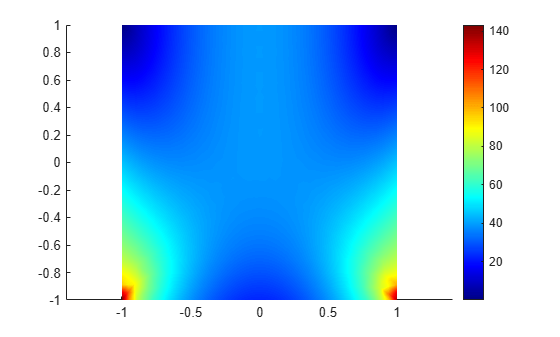Find the fundamental (lowest) mode of a 2-D cantilevered beam, assuming prevalence of the plane-stress condition.

Specify the following geometric and structural properties of the beam, along with a unit plane-stress thickness.

```length = 5; height = 0.1; E = 3E7; nu = 0.3; rho = 0.3/386;```

Create a model plane-stress model, assign a geometry, and generate a mesh.

```structuralmodel = createpde('structural','modal-planestress'); gdm = [3;4;0;length;length;0;0;0;height;height]; g = decsg(gdm,'S1',('S1')'); geometryFromEdges(structuralmodel,g);```

Define a maximum element size (five elements through the beam thickness).

```hmax = height/5; msh=generateMesh(structuralmodel,'Hmax',hmax);```

Specify the structural properties and boundary constraints.

```structuralProperties(structuralmodel,'YoungsModulus',E, ... 'MassDensity',rho, ... 'PoissonsRatio',nu); structuralBC(structuralmodel,'Edge',4,'Constraint','fixed');```

Compute the analytical fundamental frequency (Hz) using the beam theory.

```I = height^3/12; analyticalOmega1 = 3.516*sqrt(E*I/(length^4*(rho*height)))/(2*pi)```
```analyticalOmega1 = 126.9498 ```

Specify a frequency range that includes an analytically computed frequency and solve the model.

`modalresults = solve(structuralmodel,'FrequencyRange',[0,1e6])`
```modalresults = ModalStructuralResults with properties: NaturalFrequencies: [32x1 double] ModeShapes: [1x1 FEStruct] Mesh: [1x1 FEMesh] ```

The solver finds natural frequencies and modal displacement values at nodal locations. To access these values, use `modalresults.NaturalFrequencies` and `modalresults.ModeShapes`.

`modalresults.NaturalFrequencies/(2*pi)`
```ans = 32×1 105 × 0.0013 0.0079 0.0222 0.0433 0.0711 0.0983 0.1055 0.1462 0.1930 0.2455 ⋮ ```
`modalresults.ModeShapes`
```ans = FEStruct with properties: ux: [6511x32 double] uy: [6511x32 double] Magnitude: [6511x32 double] ```

Plot the y-component of the solution for the fundamental frequency.

```pdeplot(structuralmodel,'XYData',modalresults.ModeShapes.uy(:,1)) title(['First Mode with Frequency ', ... num2str(modalresults.NaturalFrequencies(1)/(2*pi)),' Hz']) axis equal```Solve an electromagnetic problem and find the electric potential and field distribution for a 2-D geometry representing a plate with a hole.

Create an electromagnetic model for electrostatic analysis.

`emagmodel = createpde('electromagnetic','electrostatic');`

Import and plot the geometry representing a plate with a hole.

```importGeometry(emagmodel,'PlateHolePlanar.stl'); pdegplot(emagmodel,'EdgeLabels','on')```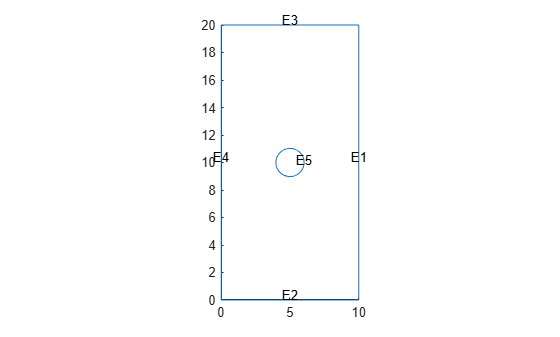Specify the vacuum permittivity in the SI system of units.

`emagmodel.VacuumPermittivity = 8.8541878128E-12;`

Specify the relative permittivity of the material.

`electromagneticProperties(emagmodel,'RelativePermittivity',1);`

Apply the voltage boundary conditions on the edges framing the rectangle and the circle.

```electromagneticBC(emagmodel,'Voltage',0,'Edge',1:4); electromagneticBC(emagmodel,'Voltage',1000,'Edge',5);```

Specify the charge density for the entire geometry.

`electromagneticSource(emagmodel,'ChargeDensity',5E-9);`

Generate the mesh.

`generateMesh(emagmodel);`

Solve the model.

`R = solve(emagmodel)`
```R = ElectrostaticResults with properties: ElectricPotential: [1218x1 double] ElectricField: [1x1 FEStruct] ElectricFluxDensity: [1x1 FEStruct] Mesh: [1x1 FEMesh] ```

Plot the electric potential and field.

```pdeplot(emagmodel,'XYData',R.ElectricPotential, ... 'FlowData',[R.ElectricField.Ex ... R.ElectricField.Ey]) axis equal```Plot the `p,e,t` mesh. Display the solution using 2-D and 3-D colored plots.

Create the geometry, mesh, boundary conditions, PDE coefficients, and solution.

```[p,e,t] = initmesh('lshapeg'); u = assempde('lshapeb',p,e,t,1,0,1);```

Plot the mesh.

`pdeplot(p,e,t)`Plot the solution as a 2-D colored plot.

`pdeplot(p,e,t,'XYData',u)`Plot the solution as a 3-D colored plot.

`pdeplot(p,e,t,'XYData',u,'ZData',u)`## Input Arguments

collapse all

Model object, specified as a `PDEModel` object, `ThermalModel` object, `StructuralModel` object, or `ElectromagneticModel` object.

Example: `model = createpde(1)`

Example: ```thermalmodel = createpde('thermal','steadystate')```

Example: ```structuralmodel = createpde('structural','static-solid')```

Example: ```emagmodel = createpde('electromagnetic','magnetostatic')```

Mesh object, specified as the `Mesh` property of a `PDEModel` object or as the output of `generateMesh`.

Example: `model.Mesh`

Nodal coordinates, specified as a 2-by-NumNodes matrix. NumNodes is the number of nodes.

Element connectivity matrix in terms of the node IDs, specified as a 3-by-NumElements or 6-by-NumElements matrix. Linear meshes contain only corner nodes. For linear meshes, the connectivity matrix has three nodes per 2-D element. Quadratic meshes contain corner nodes and nodes in the middle of each edge of an element. For quadratic meshes, the connectivity matrix has six nodes per 2-D element.Mesh points, specified as a 2-by-`Np` matrix of points, where `Np` is the number of points in the mesh. For a description of the (`p`,`e`,`t`) matrices, see Mesh Data as [p,e,t] Triples.

Typically, you use the `p`, `e`, and `t` data exported from the PDE Modeler app, or generated by `initmesh` or `refinemesh`.

Example: `[p,e,t] = initmesh(gd)`

Data Types: `double`

Mesh edges, specified as a `7`-by-`Ne` matrix of edges, where `Ne` is the number of edges in the mesh. For a description of the (`p`,`e`,`t`) matrices, see Mesh Data as [p,e,t] Triples.

Typically, you use the `p`, `e`, and `t` data exported from the PDE Modeler app, or generated by `initmesh` or `refinemesh`.

Example: `[p,e,t] = initmesh(gd)`

Data Types: `double`

Mesh triangles, specified as a `4`-by-`Nt` matrix of triangles, where `Nt` is the number of triangles in the mesh. For a description of the (`p`,`e`,`t`) matrices, see Mesh Data as [p,e,t] Triples.

Typically, you use the `p`, `e`, and `t` data exported from the PDE Modeler app, or generated by `initmesh` or `refinemesh`.

Example: `[p,e,t] = initmesh(gd)`

Data Types: `double`

### Name-Value Pair Arguments

Specify optional comma-separated pairs of `Name,Value` arguments. `Name` is the argument name and `Value` is the corresponding value. `Name` must appear inside quotes. You can specify several name and value pair arguments in any order as `Name1,Value1,...,NameN,ValueN`.

Example: `pdeplot(model,'XYData',u,'ZData',u)`

When you use a `PDEModel` object, `pdeplot(model,'XYData',u,'ZData',u)` sets surface plot coloring to the solution `u`, and sets the heights for a 3-D plot to `u`. Here `u` is a `NodalSolution` property of the PDE results returned by `solvepde` or `solvepdeeig`.

When you use a `[p,e,t]` representation, `pdeplot(p,e,t,'XYData',u,'ZData',u)` sets surface plot coloring to the solution `u` and sets the heights for a 3-D plot to the solution `u`. Here `u` is a solution returned by a legacy solver, such as `assempde`.

Tip

Specify at least one of the `FlowData` (vector field plot), `XYData` (colored surface plot), or `ZData` (3-D height plot) name-value pairs. Otherwise, `pdeplot` plots the mesh with no data.

Data Plots

collapse all

Colored surface plot data, specified as the comma-separated pair consisting of `'XYData'` and a vector. If you use a `[p,e,t]` representation, specify data for points in a vector of length `size(p,2)`, or specify data for triangles in a vector of length `size(t,2)`.

• Typically, you set `XYData` to the solution `u`. The `pdeplot` function uses `XYData` for coloring both 2-D and 3-D plots.

• `pdeplot` uses the colormap specified in the `ColorMap` name-value pair, using the style specified in the `XYStyle` name-value pair.

• When the `Contour` name-value pair is `'on'`, `pdeplot` also plots level curves of `XYData`.

• `pdeplot` plots the real part of complex data.

To plot the `k`th component of a solution to a PDE system, extract the relevant part of the solution. For example, when using a `PDEModel` object, specify:

```results = solvepde(model); u = results.NodalSolution; % each column of u has one component of u pdeplot(model,'XYData',u(:,k)) % data for column k```

When using a `[p,e,t]` representation, specify:

```np = size(p,2); % number of node points uk = reshape(u,np,[]); % each uk column has one component of u pdeplot(p,e,t,'XYData',uk(:,k)) % data for column k```

Example: `'XYData',u`

Data Types: `double`

Coloring choice, specified as the comma-separated pair consisting of `'XYStyle'` and `'interp'`, `'off'`, or `'flat'`.

• `'off'` — No shading, only mesh is displayed.

• `'flat'` — Each triangle in the mesh has a uniform color.

• `'interp'` — Plot coloring is smoothly interpolated.

The coloring choice relates to the `XYData` name-value pair.

Example: `'XYStyle','flat'`

Data Types: `char` | `string`

Data for the 3-D plot heights, specified as the comma-separated pair consisting of `'ZData'` and a matrix. If you use a `[p,e,t]` representation, provide data for points in a vector of length `size(p,2)` or data for triangles in a vector of length `size(t,2)`.

• Typically, you set `ZData` to `u`, the solution. The `XYData` name-value pair sets the coloring of the 3-D plot.

• The `ZStyle` name-value pair specifies whether the plot is continuous or discontinuous.

• `pdeplot` plots the real part of complex data.

To plot the `k`th component of a solution to a PDE system, extract the relevant part of the solution. For example, when using a `PDEModel` object, specify:

```results = solvepde(model); u = results.NodalSolution; % each column of u has one component of u pdeplot(model,'XYData',u(:,k),'ZData',u(:,k)) % data for column k```

When using a `[p,e,t]` representation, specify:

```np = size(p,2); % number of node points uk = reshape(u,np,[]); % each uk column has one component of u pdeplot(p,e,t,'XYData',uk(:,k),'ZData',uk(:,k)) % data for column k```

Example: `'ZData',u`

Data Types: `double`

3-D plot style, specified as the comma-separated pair consisting of `'ZStyle'` and one of these values:

• `'off'` — No 3-D plot.

• `'discontinuous'` — Each triangle in the mesh has a uniform height in a 3-D plot.

• `'continuous'` — 3-D surface plot is continuous.

If you use `ZStyle` without specifying the `ZData` name-value pair, then `pdeplot` ignores `ZStyle`.

Example: `'ZStyle','discontinuous'`

Data Types: `char` | `string`

Data for the quiver plot, specified as the comma-separated pair consisting of `'FlowData'` and an `M`-by-`2` matrix, where `M` is the number of mesh nodes. `FlowData` contains the x and y values of the field at the mesh points.

When you use a `PDEModel` object, set `FlowData` as follows:

```results = solvepde(model); gradx = results.XGradients; grady = results.YGradients; pdeplot(model,'FlowData',[gradx grady])```

When you use a `[p,e,t]` representation, set `FlowData` as follows:

```[gradx,grady] = pdegrad(p,t,u); % Calculate gradient pdeplot(p,e,t,'FlowData',[gradx;grady])```

When you use `ZData` to represent a 2-D PDE solution as a 3-D plot and you also include a quiver plot, the quiver plot appears in the z = 0 plane.

`pdeplot` plots the real part of complex data.

Example: `'FlowData',[ux uy]`

Data Types: `double`

Indicator to show the quiver plot, specified as the comma-separated pair consisting of `'FlowStyle'` and `'arrow'` or `'off'`. Here, `'arrow'` displays the quiver plot specified by the `FlowData` name-value pair.

Example: `'FlowStyle','off'`

Data Types: `char` | `string`

Indicator to convert the mesh data to x-y grid before plotting, specified as the comma-separated pair consisting of `'XYGrid'` and `'off'` or `'on'`.

Note

This conversion can change the geometry and lessen the quality of the plot.

By default, the grid has about `sqrt(size(t,2))` elements in each direction.

Example: `'XYGrid','on'`

Data Types: `char` | `string`

Customized x-y grid, specified as the comma-separated pair consisting of `'GridParam'` and a matrix `[tn;a2;a3]`. For example:

```[~,tn,a2,a3] = tri2grid(p,t,u,x,y); pdeplot(p,e,t,'XYGrid','on','GridParam',[tn;a2;a3],'XYData',u)```

For details on the grid data and its `x` and `y` arguments, see `tri2grid`. The `tri2grid` function does not work with `PDEModel` objects.

Example: `'GridParam',[tn;a2;a3]`

Data Types: `double`

Mesh Plots

collapse all

Node labels, specified as the comma-separated pair consisting of `'NodeLabels'` and `'off'` or `'on'`.

`pdeplot` ignores `NodeLabels` when you use it with `ZData`.

Example: `'NodeLabels','on'`

Data Types: `char` | `string`

Element labels, specified as the comma-separated pair consisting of `'ElementLabels'` and `'off'` or `'on'`.

`pdeplot` ignores `ElementLabels` when you use it with `ZData`.

Example: `'ElementLabels','on'`

Data Types: `char` | `string`

Structural Analysis Plots

collapse all

Data for plotting the deformed shape for a structural analysis model, specified as the comma-separated pair consisting of `'Deformation'` and the `Displacement` property of the `StaticStructuralResults` object.

In an undeformed shape, center nodes in quadratic meshes are always added at half-distance between corners. When you plot a deformed shape, the center nodes might move away from the edge centers.

Example: `'Deformation',structuralresults.Displacement`

Scaling factor for plotting the deformed shape, specified as the comma-separated pair consisting of `'DeformationScaleFactor'` and a real number. Use this argument with the `Deformation` name-value pair. The default value is defined internally, based on the dimensions of the geometry and the magnitude of the deformation.

Example: `'DeformationScaleFactor',100`

Data Types: `double`

Annotations and Appearance

collapse all

Indicator to include a color bar, specified as the comma-separated pair consisting of `'ColorBar'` and `'on'` or `'off'`. Specify `'on'` to display a bar giving the numeric values of colors in the plot. For details, see `colorbar`. The `pdeplot` function uses the colormap specified in the `ColorMap` name-value pair.

Example: `'ColorBar','off'`

Data Types: `char` | `string`

Colormap, specified as the comma-separated pair consisting of `'ColorMap'` and a value representing a built-in colormap, or a colormap matrix. For details, see `colormap`.

`ColorMap` must be used with the `XYData` name-value pair.

Example: `'ColorMap','jet'`

Data Types: `double` | `char` | `string`

Indicator to show the mesh, specified as the comma-separated pair consisting of `'Mesh'` and `'on'` or `'off'`. Specify `'on'` to show the mesh in the plot.

Example: `'Mesh','on'`

Data Types: `char` | `string`

Title of plot, specified as the comma-separated pair consisting of `'Title'` and a character vector.

Example: `'Title','Solution Plot'`

Data Types: `char` | `string`

Surface transparency for 3-D geometry, specified as the comma-separated pair consisting of `'FaceAlpha'` and a real number from `0` through `1`. The default value `1` indicates no transparency. The value `0` indicates complete transparency.

Example: `'FaceAlpha',0.5`

Data Types: `double`

Indicator to plot level curves, specified as the comma-separated pair consisting of `'Contour'` and `'off'` or `'on'`. Specify `'on'` to plot level curves for the `XYData` data. Specify the levels with the `Levels` name-value pair.

Example: `'Contour','on'`

Data Types: `char` | `string`

Levels for contour plot, specified as the comma-separated pair consisting of `'Levels'` and a positive integer or a vector of level values.

• Positive integer — Plot `Levels` as equally spaced contours.

• Vector — Plot contours at the values in `Levels`.

To obtain a contour plot, set the `Contour` name-value pair to `'on'`.

Example: `'Levels',16`

Data Types: `double`

## Output Arguments

collapse all

Handles to graphics objects, returned as a vector.

## More About

collapse all

### Quiver Plot

A quiver plot is a plot of a vector field. It is also called a flow plot.

Arrows show the direction of the field, with the lengths of the arrows showing the relative sizes of the field strength. For details on quiver plots, see `quiver`.

## See Also

Introduced before R2006a

## SupportGet trial now